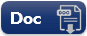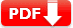# Fractions and Decimals Practice Test: Question Answers

A fraction is either a proper fraction or an improper fraction. A proper fraction is a number representing a part of a whole. This whole may be a single object or a group of objects. An improper fraction is a number in which numerator is greater than the denominator. A mixed fraction is a combination of a natural number and a proper fraction.

 Test Name Fractions and Decimals Test Prep Type of Question Multiple Choice Question Answers Subject Math Total Question 10 Test Type Sample / Mock Test Editable & Printable PDF / Doc YES (Download link is given below) Available of Answers YES Difficulty Level Pre-Algebra/Elementary Algebra

### Fractions and Decimals Practice Test:

Test Set: 1

 1. Sophia is dividing 3 \frac14 kg of sweets equally among her seven friends. How much does each friend receive? 🔘   \frac14 kg 🔘  \frac24 kg 🔘   \frac34 kg 🔘  \frac4{14} kg 🔘   \frac{1} {24} kg
 2. If   \frac34  of a number is 12, then the number is 🔘 8 🔘 16 🔘 18 🔘 28 🔘 32
 3. Given that 0 < p < q < r < s and p, q, r, s are integers, which of the following is the smallest? 🔘   \frac{p + q} {r + s} 🔘   \frac{p + s} {q + r} 🔘   \frac{q + s} {p + r} 🔘   \frac{r + s} {p + q} 🔘   \frac{q + r} {s + p}
Answer: A   \frac{p + q} {r + s}
 4. The decimal expression for 8 USD 8 cents  (in USD) is 🔘 8.8 🔘 8.08 🔘 8.008 🔘 88.00 🔘 08.80
 5. 2.5 ÷ 1000 is equal to 🔘 0.025 🔘 0.0025 🔘 0.2500 🔘 2.50 🔘 250
 6. Which of the following has the smallest value? 🔘 0.0002 🔘   \frac{2} {1000} 🔘   \frac{(0.2)} {1000} 🔘   \frac{2} {1000} ÷ 0.01 🔘 0.200
 7. Which of the following has the largest value? 🔘   \frac{32} {0.05} 🔘   \frac{0.320} {50} 🔘   \frac{3.2} {0.05} 🔘   \frac{3.2} {50} 🔘   \frac{3.02} {0.50}
 Document Type Download Link Free Editable Doc FileFree Printable PDF File